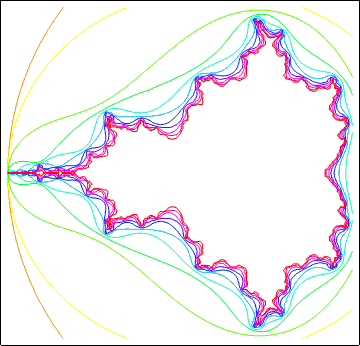# Mandelbrot Set Discussion

The Mandelbrot set is the set of complex points c that do not escape to infinity when repeatedly applying the mapping z → z2 + c, which admittedly doesn’t sound very interesting. (We might as well define f(z) = z2 + c to avoid having to type “the mapping” again and again; and this is as good a time as any to mention that we always start the iteration with f(0).)

Suppose we start with c = 0. The first iteration of f gives f(0) = 02 + 0 = 0; the next iteration gives f(f(0)) = f(0) = 0; next is f(f(f(0))) = 0, and so forth: when c is zero, all iterates of f are equal to zero.

Next, suppose we choose c = 1. This gives us

 f(0) = 02 + 1 = 1, f(1) = 12 + 1 = 2, f(2) = 22 + 1 = 5, f(5) = 52 + 1 = 26, f(26) = 262 + 1 = 677,

and so forth: the values ultimately escape to infinity.

When we choose c = -1, we get

 f(0) = 02 - 1 = -1, f(-1) = (-1)2 - 1 = 0, f(0) = 02 - 1 = -1,

so the values alternate between -1 and 0, and the iteration does not escape.

For any c, the first few iterations of f are {0, c, c + c2, c + (c + c2)2, c + (c + (c + c2)2)2, ...}, and it turns out that the boundary of the set of complex values of c that do not escape is very complicated. It can be shown that if the absolute value of an iterate of f becomes larger than 2, the iteration escapes to infinity; the following is a graph of the level curves (|fn(z)| = 2) of the successive iterates of f.```Show[
MapIndexed[
Graphics[
ContourPlot[Abs[#], {a, -2.0, 0.5}, {b, -1.2, 1.2},
ContourStyle :> Hue[#2[]/11.0],
DisplayFunction->Identity, PlotPoints->123]]&,
NestList[#^2 + a + b I &, a + b I, 10]],
DisplayFunction->\$DisplayFunction, AspectRatio->Automatic]```

The simplest way to draw filled Mandelbrot sets seems to be to compute the iterates of f, using a more or less fine grid of complex values of c, coloring each point according to the number of iterations it took for the values to escape to infinity (exceed 2, that is). (At least that‘s the way I did it here.)

Designed and rendered using Mathematica 3.0 for the Apple Macintosh and (much, much later) 7.0 for Microsoft Windows.